## Simulating Thermal Effects in Semiconductors

Thermal modeling provides data that helps you to estimate cooling requirements for your system by using the thermal ports. Some of the blocks in the Simscape™ Electrical™ Semiconductors & Converters library have thermal variants that allow you to determine device temperatures by simulating heat generation. For example, the IGBT (Ideal, Switching) block, which models a three-terminal semiconductor device, has thermal variants that can simulate the heat generated by switching events and conduction losses.

For more information on selecting the parameter values, see Improving Numerical Performance. For explanation of the relationship between the Thermal Port and Temperature Dependence settings in a block dialog box, see Electrical Behavior Depending on Temperature.

### Using the Thermal Ports

Certain Simscape Electrical blocks, such as the blocks in the Semiconductors & Converters library, contain an optional thermal port that is hidden by default. If you want to simulate the generated heat and device temperature, expose the thermal port by:

1. Double-click the block where you want to show the thermal port.

2. Set the Modeling option parameter to ```Show thermal port```.

When the thermal port is exposed, the Block Parameters window for that block contains an additional setting, Thermal Port. Which parameters are visible depend on the value you set for the Thermal network parameter:

All blocks with optional thermal ports include an optional internal thermal model to keep your diagram uncluttered.

### Specify junction and thermal case parameters

This figure shows an equivalent model of the ```Specify junction and thermal case parameters``` model for semiconductor devices.Port H corresponds to thermal port H of the block. The two Thermal Mass blocks represent the thermal mass of the device case and the thermal mass of the semiconductor junction, respectively. The Heat Flow Rate Source block (called power_dissipated in the diagram) inputs heat to the model with a value equal to the electrically generated heat from the device.

The two Conductive Heat Transfer blocks model the thermal resistances. Resistance R_JC (conductance 1/R_JC) represents the thermal resistance between junction and case. Because of this resistance, the junction will be hotter than the case under normal conditions. Resistance R_CA represents the thermal resistance between port H and the device case. If the device has no heat sink, then you should connect port H to a Temperature Source block with its temperature set to ambient conditions. If your device does have an external heat sink, then you must model the heat sink externally to the device and connect the heat sink thermal mass directly to port H.

If you choose to simulate the internal thermal network of the block through junction and thermal case parameters, these parameters are enabled:

• Junction case and case-ambient (or case-heatsink) thermal resistances, [R_JC R_CA] — A row vector [ R_JC R_CA ] of two thermal resistance values, represented by the two Conductive Heat Transfer blocks. The first value, R_JC, is the thermal resistance between the junction and the case. The second value, R_CA, is the thermal resistance between port H and the device case.

• Thermal mass parameterization — Select whether you want to parameterize the thermal masses in terms of thermal time constants (`By thermal time constants`), or specify the thermal mass values directly (`By thermal mass`). For more information, see Thermal Mass Parameterization. The default is `By thermal time constants`.

• Junction and case thermal time constants, [t_J t_C] — A row vector [ t_J t_C ] of two thermal time constant values. The first value, t_J, is the junction time constant. The second value, t_C, is the case time constant. To enable this parameter, set the Thermal mass parameterization to ```By thermal time constants```.

• Junction and case thermal masses, [M_J M_C] — A row vector [ M_J M_C ] of two thermal mass values. The first value, M_J, is the junction thermal mass. The second value, M_C, is the case thermal mass. To enable this parameter, set the Thermal mass parameterization to ```By thermal mass```.

• Junction and case initial temperatures, [T_J T_C] — A row vector [ T_J T_C ] of two temperature values. The first value, T_J, is the junction initial temperature. The second value, T_C, is the case initial temperature.

The following rules apply:

• Case thermal mass must be greater than zero.

• Junction thermal mass can only be set to zero if the junction-case resistance is also set to zero.

• If both the case and junction thermal masses are defined, but the junction-case resistance is zero, then the initial temperatures assigned to the junction and case must be identical.

### Cauer Model

This figure shows an equivalent model of the internal Cauer thermal model for semiconductor devices.If you choose to simulate the internal thermal network of the block through Cauer model, these parameters are enabled:

• Thermal resistances, [R1 R2 … Rn] — A row vector of n thermal resistance values, represented by the Cauer elements used in the thermal network. These values must all be greater than zero.

• Thermal mass parameterization — Select whether you want to parameterize the thermal masses in terms of thermal time constants (`By thermal time constants`), or specify the thermal mass values directly (`By thermal mass`). For more information, see Thermal Mass Parameterization. The default is `By thermal time constants`.

• Thermal time constants, [t1 t2 … tn] — A row vector of n thermal time constant values, where n is the number of Cauer elements used in the thermal network. The length of this vector must match the length of Thermal resistances, [R1 R2 … Rn]. These values must all be greater than zero. With this parameterization, the thermal masses are computed as ```Mi = ti/Ri```, where `Mi`, `ti` and `Ri` are the thermal mass, thermal time, and thermal resistance for the ith Cauer element. To enable this parameter, set Thermal mass parameterization to ```By thermal time constants```.

• Thermal masses, [M1 M2 … Mn] — A row vector of n thermal mass values, where n is the number of Cauer elements used in the thermal network. These values must all be greater than zero. To enable this parameter, set Thermal mass parameterization to ```By thermal mass```.

• Thermal masses initial temperatures, [T1 T2 … Tn] — A row vector of temperature values. It corresponds to the temperature drop across each thermal capacity in the model.

### Cauer Model Parameterized with Foster Coefficients

The datasheets of semiconductor devices often use Foster coefficients to specify the thermal model. However, Cauer thermal models are more useful as you can extend them with additional thermal components such as heatsinks, radiation, and convection elements.

If you want to model the internal thermal network of the semiconductor device with Foster coefficients, set the Thermal network parameter of the block to `Cauer model parameterized with Foster coefficients`.

Note

This thermal network is implemented as a Cauer thermal network, but it behaves exactly as a Foster model implementation using the same parameters.

This figure shows the equivalent thermal model with a Cauer implementation, where each Cauer Thermal Model block has the Parameterize Cauer model using Foster coefficient data parameter selected.This option allows you to connect a heatsink and other extensions to the thermal network while parameterizing the thermal model with Foster coefficients.

If you choose to simulate the internal thermal network of the block through a Cauer model parameterized with Foster coefficients, these parameters are enabled:

• Thermal resistances, [R1 R2 … Rn] — A row of n thermal resistance values, represented by the Cauer elements used in the thermal network. These values must all be greater than zero.

• Thermal mass parameterization — Select whether you want to parameterize the thermal masses in terms of thermal time constants (`By thermal time constants`), or specify the thermal mass values directly (`By thermal mass`). For more information, see Thermal Mass Parameterization. The default is `By thermal time constants`.

• Thermal time constants, [t1 t2 … tn] — A row vector of n thermal time constant values, where n is the number of Cauer elements used in the thermal network. The length of this vector must match the length of Thermal resistances, [R1 R2 … Rn]. These values must all be greater than zero. With this parameterization, the thermal masses are computed as ```Mi = ti/Ri```, where `Mi`, `ti` and `Ri` are the thermal mass, thermal time, and thermal resistance for the ith Foster element. To enable this parameter, set Thermal mass parameterization to ```By thermal time constants```.

• Thermal masses, [M1 M2 … Mn] — A row vector of n thermal mass values, where n is the number of Cauer elements used in the thermal network. These values must all be greater than zero. To enable this parameter, set Thermal mass parameterization to ```By thermal mass```.

• Initial node temperatures, [T1 T2 … Tn] — A row vector of absolute temperature values of each node starting from the junction.

### External Model

If you want to model the thermal network of a semiconductor block externally to the block itself, set the Thermal network parameter to `External`. This figure shows the equivalent model of the internal thermal model for semiconductor devices.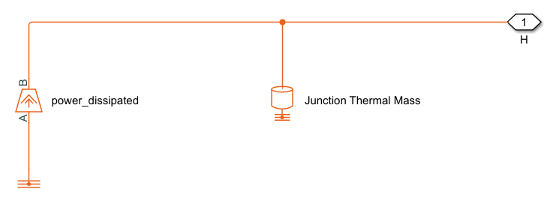Port H corresponds to thermal port H of the block. The Heat Flow Rate Source block (called power_dissipated in the diagram) represents the total dissipated power in the block. The dissipated power is output as heat flow to the H node. Similar to the Foster thermal model, you need to connect a thermal source or additional thermal components to the H node so that the heat has somewhere to flow.

If you choose to simulate the internal thermal network of the block externally, the Junction thermal mass parameter is enabled.

### Thermal Mass Parameterization

If you need to estimate thermal masses, there are two parameterization options:

• `By thermal time constants` — Parameterize the thermal masses in terms of thermal time constants. This is the default.

• `By thermal mass` — Specify the thermal mass values directly.

For the `Cauer model (junction and case)`, the thermal time constants t_J and t_C are defined as follows:

t_J = M_J · R_JC

t_C = M_C · R_CA

where M_J and M_C are the junction and case thermal masses, respectively, R_JC is the thermal resistance between junction and case, and R_CA is the thermal resistance between port H and the device case.

For the `Foster model`, the thermal time constant, ti, is defined as follows for the ith Foster element:

ti = Mi· Ri,

where Mi and Ri are the thermal mass and the thermal resistance of the ith Foster element, respectively.

You can determine the case-time constant by experimental measurement. If data is not available for the junction-time constant, you can either omit the constant and set the junction-case resistance to zero, or you can set the junction-time constant to a typical value of one-tenth of the case-time constant. Alternatively, you can estimate thermal masses based on the device dimensions and averaged material-specific heats.

### Electrical Behavior Depending on Temperature

For blocks with optional thermal ports, there are two simulation options:

• Simulate the generated heat, device temperature, and the effect of temperature on the electrical equations.

• Simulate the generated heat and device temperature, but do not include effect of temperature on the electrical equations. Use this option when the impact of temperature on the electrical equations is small for the temperature range that you are simulating, or where the primary task of the simulation is to capture the heat generated to support system-level design.

The thermal port and the Thermal Port setting of the Block Parameters window let you simulate the generated heat and device temperature. For blocks with a Temperature Dependence setting, it is possible to simulate the impact of the junction temperature on the electrical characteristics. The Temperature Dependence setting lets you model the effect that the temperature of the semiconductor junction has on the electrical equations. Therefore:

• To simulate all of the temperature effects, show the block’s thermal port and, if the block has a Temperature Dependence setting, set the Parameterization parameter to one of the provided options, for example, ```Use an I-V data point at second measurement temperature```.

• To simulate only the generated heat and device temperature, show the thermal port of the block and, in the Temperature Dependence settings, set Parameterization to ```None — Simulate at parameter measurement temperature```.

### Improving Numerical Performance

Set realistic values for thermal masses and resistances. Otherwise, junction temperatures can become extreme, and out of range for valid results, which can manifest as numerical difficulties during simulation. You can test if numerical difficulties are a result of unrealistic thermal values by turning off the temperature dependence for the electrical equations. Open the Block Parameters window and, in the Temperature Dependence settings, set Parameterization to ```None — Simulate at parameter measurement temperature```.

The thermal time constants are generally much slower than electrical time constants, so the thermal aspects of your model are unlikely to dictate the maximum fixed time step you can simulate at (for example, for hardware-in-the-loop simulations). However, if you need to remove detail (for example, to speed up simulation), the junction-thermal mass time constant is typically an order of magnitude faster than the case-thermal mass time constant. You can remove the effect of the junction-thermal mass by setting the junction-thermal mass and junction-case thermal resistance to zero.

### Model Thermal Losses for a Rectifier

#### Model Heat Transfer for a Single Rectifier Diode

To model and measure heat transfer as a function of the thermal characteristics of a semiconductor, connect a Cauer-based thermal network parameterized with Foster coefficients, and a temperature sensor to a block with a thermal port.

1. Open the model. At the MATLAB® command prompt, enter:

`ee_rectifier_diodes`

The model contains a three-phase rectifier that includes six Diode blocks.

2. Select a thermal modeling option for the Diode1 block by double-clicking the block and setting the Modeling option parameter to ```Show thermal port```.

3. Open the Diode1 block.

1. In the Thermal Port settings, set Thermal network to `External`.

2. In the Initial Targets settings, select Junction temperature, and set Priority to `High` and Value to `300` `K`.

4. Add a Simscape Electrical block that represents the heat flow between the diode and the environment. Open the Simulink® Library browser, click Simscape > Electrical > Passive > Thermal, and add a Cauer Thermal Model block to the model.

5. Open the Cauer Thermal Model block and modify these parameters:

1. In the Parameters settings:

• Select the Parameterize Cauer model using Foster coefficient data parameter.

• Set Thermal resistance data (Foster) to ```[ 0.00311 0.008493 0.00252 0.00288 ]``` `K/W`.

• Set Thermal time constant data (Foster) to ```[ 0.0068 0.0642 0.3209 2.0212 ]``` `s`.

2. In the Initial Targets settings:

• Select Vector of thermal mass temperatures.

• Set Priority to `High`.

6. Add these blocks to represent the ambient temperature as a constant by using an ideal temperature source.

1. From the Simulink Library browser, open the Simscape > Foundation Library > Thermal > Thermal Sources library and add a Controlled Temperature Source block.

2. From the Simscape > Foundation Library > Thermal > Thermal Elements library, add a Thermal Reference block.

3. From the Simscape > Foundation Library > Physical Signals > Sources library, add a PS Constant block. For the Constant parameter, specify a value of `300` and select `K` for the unit.

7. Add these blocks to measure and display the temperature of Diode1:

1. From the Simulink Library browser, open the Simscape > Foundation Library > Thermal > Thermal Sensors library and add a Temperature Sensor block.

2. From the Simscape > Utilities library, add a PS-Simulink Converter block. For the Output signal unit parameter, select `K`.

3. From the Simulink > Sinks library, and add a Scope block.

8. Arrange and connect the blocks as shown in the figure.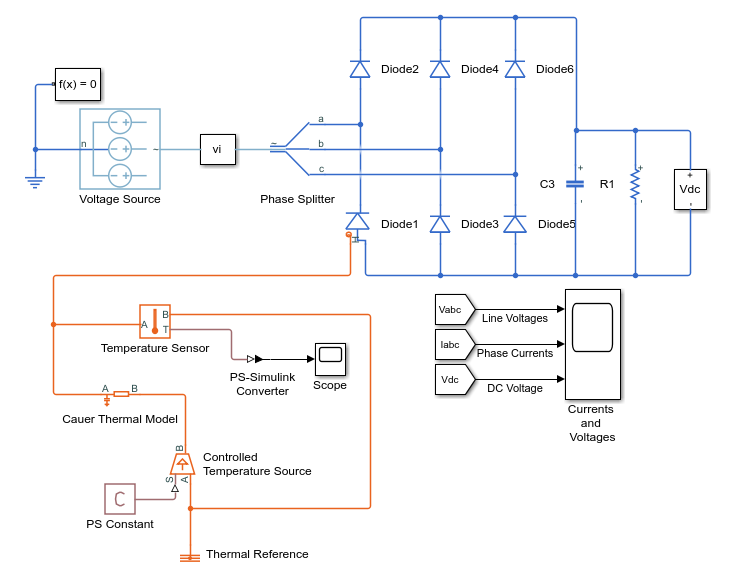9. Label the signal from the PS-Simulink Converter block to the Scope block by double-clicking the line between the blocks and entering ```Temp (K)```.

10. Simulate the model.

11. To see the temperature data, open the Scope block.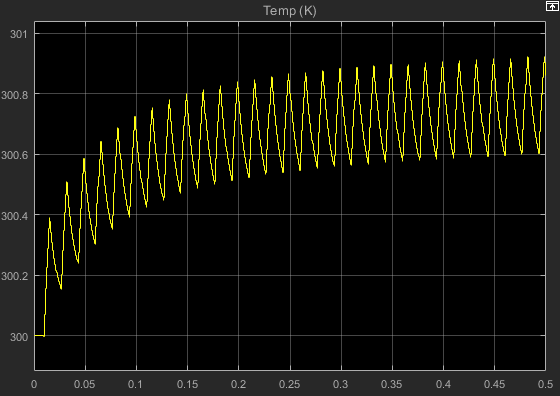The temperature of Diode1 fluctuates over a temperature range of 0.3 K as it increases from the initial value of 300 K to a settling point of 300.6–300.9 K toward the end of the simulation.

#### Model Heat Transfer for All Rectifier Diodes

To see the total heat generated by all of the semiconductors in the rectifier, use data logging and the Simscape Results Explorer.

1. To enable the thermal ports on all the rectifier diodes, select thermal modeling options for the Diode2, Diode3, Diode4, Diode5, and Diode6 blocks by double-clicking the blocks and setting the Modeling option parameter to ```Show thermal port```.

2. Open the Diode2, Diode3, Diode4, Diode5, and Diode6 blocks.

1. In the Thermal Port settings, set Thermal network to `External`.

2. In the Initial Targets settings, select Junction temperature, and set Priority to `High` and Value to `300` `K`.

3. Add blocks to measure the heat transfer for each diode by creating a Cauer thermal model subsystem.

1. Make a copy of this group of blocks:

• Cauer Thermal Model

• Controlled Temperature Source

• PS Constant

• Thermal Reference

2. Arrange and connect the copied blocks as shown in the figure.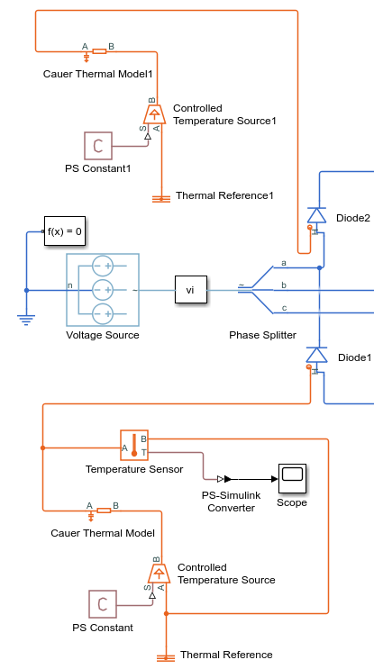3. Create a subsystem from the copied blocks and rename the subsystem as Cauer_D2. For more information, see Group Blocks into Subsystems.

4. Open the Cauer_D2 subsystem. Open the Conn1 block, and for the Port location on the parent subsystem parameter, select `Right`.5. Make four copies of the Cauer_D2 subsystem. Attach one subsystem to each of the remaining Diode blocks and rename the subsystems as Cauer_D3 through Cauer_D6 to match the Diode3 through Diode6 block names.

4. Simulate the model.

5. View the results using the Simscape Results Explorer.

1. In the model window, in the text under Three-Phase Rectifier, click Explore simulation results.

2. To display the temperature data for Diode1, in the Simscape Results Explorer window, expand the Diode1 > H node and click T.

3. To display the DC voltage in a separate plot, expand the Sensing Vdc > Voltage Sensor node, press , and click V.

4. To display the temperature data for all the diodes, expand the Diode2 > H node, press , and click T. Repeat the process for Diode3 through Diode6.

5. To overlay the temperature data in single plot, in the Simscape Results Explorer window, above the tree-node window, click the options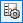button. In the Options dialog box, for Plot signals, select `Overlay`. To accept the change, click . Click and drag the legend down to see the temperature data clearly.The temperature profile for each diode lags, in succession, behind the temperature profile of Diode1. For each diode, the temperature also rises and settles along the same values as the temperature profile for Diode1. The data indicate that, because of the lagging behavior of the individual diode temperatures, the temperature of the rectifier rises and settles along the same temperature profile as the diodes, but with less fluctuation.